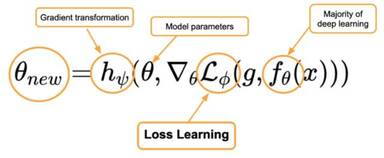## What Is Meta-Learning via Learned Losses (with Python Code)

Source – https://analyticsindiamag.com/

Facebook AI Research (FAIR) research on meta-learning has majorly classified into two types:  First, methods that can learn representation for generalization. Second, methods that can optimize models. We have thoroughly discussed the type first in our previous article MBIRL. For this post, we are going to give a brief introduction to the second type. Last month, at the International Conference on Pattern Recognition, {ICPR}, Italy, January 10-15, 2021, a group of researchers: S. Bechtle,  A. Molchanov, Y. Chebotar, E. Grefenstette, L. Righetti, G. S. Sukhatme, F. Meier submitted a research paper focussing on the automation of “meta-training” processing: Meta Learning via Learned Loss.

Motivation Behind ML3

In meta-learning, the goal is to efficiently optimize the function fθ  which can be a regressor or classifier that finds the optimal value of θL is the loss function and h is the gradient transform. The majority of the work in deep learning is associated with learning the function directly from data and some meta-learning work focuses on the parameter updation. In ML3 approach, the authors have targeted loss learning. Loss functions are architecture independent and widely used for learning problems so learning a loss function doesn’t require any engineering and optimization and allows the addition of extra information during meta-training.

The key idea of the proposed framework is to develop a pipeline for meta-training that not only can optimize the performance of the model but also generalize for different tasks and model architectures. The proposed framework of learning loss functions efficiently optimize the models for new tasks. The main contribution of the ML3 framework are :

i) It is capable of learning adaptive, high-dimensional functions via back propagation and gradient descent.

ii) The given framework is very flexible as it is capable of storing additional information at the meta-train time and provides generalization by solving regression, classification, model-based reinforcement learning, model-free reinforcement learning.

The Model Architecture of ML3

The task of learning a loss function is based on a bi-level optimization technique i.e., it contains two optimization loops: inner and outer. The inner loop is responsible for training the model or optimizee with gradient descent by using the loss function learners meta-loss function and the outer loop optimized the meta-loss function by minimizing the task loss i.e., regression or classification or reinforcement learning loss.

The process contains a function parameterized by θ that takes a variable x and outputs y. It also learns meta-loss network M parameterized by Φ  that takes the input and output of function f and together with task-specific information (for example ground truth label for regression or classification, final position in MBIRL or the sample reward from model-free reinforcement learning problems) and outputs the meta- loss function L parameterized by both Φ and θ

So, to update function f, compute the gradient of Meta Loss L with respect to θ and update the gradient using the learned loss function, as shown below :

Now, to update M, the loss network, formulate a task-specific loss that compares the output of the currently optimal with the target information since is updated with L, the task is also functional Φ and perform gradient update on Φ to optimize M. This architecture finally forms a fully differential loss learning framework used for training.

To use the learning loss  at Test time, directly update by taking the gradient of learned loss L with respect to the parameters of f.

Applications of ML3

1. Regression problems.
2. Classification problems.
3. Shaping Loss during training e.g., Covexifying Loss, exploration signal. ML3 provides a possibility to add additional information during meta-training.
4. Model-based Reinforcement Learning.
5. Model-free Reinforcement Learning.

Requirements & Installation

1. Python=3.7
2. Clone the Github repository via git.
3. Install all the dependencies of ML3 via :

Paper Experiment Demos

This section contains different experiments mentioned in the research paper.

A. Loss Learning for Regression

1. Run Sin function regression experiment by code below:
1. Now, you can visualize the results by the following code:

2.1 Import the required libraries, packages and modules and specify the path to the saved data during meta-training. The code snippet is available here.

2.2 Load the saved data during the experiment.

2.3 Visualize the performance of the meta loss when used to optimize the meta training tasks, as a function of (outer) meta training iterations.

2.4 Evaluating learned meta loss networks on test tasks. Plot the performance of the final meta loss network when used to optimize the new test tasks at meta test time. Here the x-axis represents the number of gradient descent steps. The code snippet is available here.

C. Learning with extra information at the meta-train time

This demo shows how we can add extra information during meta training in order to shape the loss function. For experiment purposes, we have taken the example of sin function. Now, with the code, the script requires two arguments, first one is train\test, the 2nd one indicates whether to use extra information by setting True\False (with\without extra info).

1. For training, the code is given below
2. To test the loss with extra information run:
1. For comparison purposes, we have repeated the above two steps with argument as False. The full code is available here.
2. Comparison of results via visualization.

Similarly, the research experiment for meta learning the loss with an additional goal in the mountain car experiment run can be done. The code lines is available here.

EndNotes

In this write-up we have given an overview Meta Learning via Learned Loss(ML3), a gradient-based bi-level optimization algorithm which is capable of learning any parametric loss function as long as the output is differential with respect to its parameters. These learned loss functions can be used to efficiently optimize models for new tasks.

Note : All the figures/images except the output of the code are taken from official sources of ML3.

• Colab Notebook ML3 Demo

Official Code, Documentation & Tutorial are available at:

• Github
• Website
• Research Paper